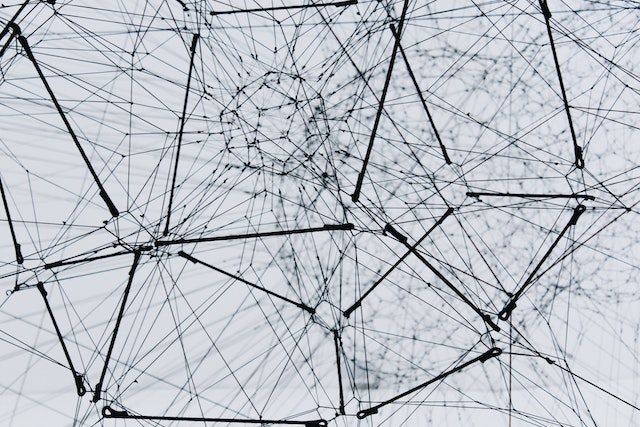# Number Classification

Neural NetworkHandwritten Number Recognition. This app classifies the number drawn on the screen into a digit. A recurrent convolutional neural network was trained on the MNIST handwritten digit dataset with TensorFlow in python. Model was imported in JavaScript with TensorFlow.js.

Features

Convolutional Neural Network: Model created in python with TensorFlow and imported via TensorFlow.js